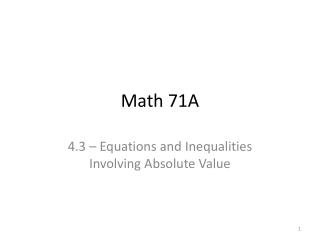DownloadDownload PresentationMath 71A

# Math 71A

Télécharger la présentation## Math 71A

- - - - - - - - - - - - - - - - - - - - - - - - - - - E N D - - - - - - - - - - - - - - - - - - - - - - - - - - -
##### Presentation Transcript

1. Math 71A 4.3 – Equations and Inequalities Involving Absolute Value

2. Equations Involving Absolute Value Here’s an example of an absolute value equation: What are the solutions to the above equation?

3. Equations Involving Absolute Value Here’s an example of an absolute value equation: What are the solutions to the above equation? and

4. Equations Involving Absolute Value Ex 1.Solve and check: Ex 2.Solve:

5. Equations Involving Absolute Value Ex 3.What the solution set of?

6. Equations Involving Absolute Value Ex 3.What the solution set of? positive negative

7. Equations Involving Absolute Value Ex 3.What the solution set of? positive negative Solution set:

8. Equations Involving Absolute Value Ex 4.Solve:

9. Solving Inequalities Involving Absolute Value Ex 5.What is the solution set of ?

10. Solving Inequalities Involving Absolute Value Ex 5.What is the solution set of ?

11. Solving Inequalities Involving Absolute Value Ex 5.What is the solution set of ?

12. Solving Inequalities Involving Absolute Value Ex 5.What is the solution set of ?

13. Solving Inequalities Involving Absolute Value Ex 6.What is the solution set of ?

14. Solving Inequalities Involving Absolute Value Ex 6.What is the solution set of ?

15. Solving Inequalities Involving Absolute Value Ex 6.What is the solution set of ?

16. Solving Inequalities Involving Absolute Value Ex 6.What is the solution set of ?

17. Solving Inequalities Involving Absolute Value In general, if □ represents an algebraic expression (like ) and is a positive #, then… …solutions of are the #’s that satisfy …solutions of are the #’s that satisfy or

18. Solving Inequalities Involving Absolute Value In general, if □ represents an algebraic expression (like ) and is a positive #, then… …solutions of are the #’s that satisfy …solutions of are the #’s that satisfy or

19. Solving Inequalities Involving Absolute Value In general, if □ represents an algebraic expression (like ) and is a positive #, then… …solutions of are the #’s that satisfy …solutions of are the #’s that satisfy or

20. Solving Inequalities Involving Absolute Value In general, if □ represents an algebraic expression (like ) and is a positive #, then… …solutions of are the #’s that satisfy …solutions of are the #’s that satisfy or (Note: the above rules work with and as well)

21. Solving Inequalities Involving Absolute Value Ex 7.Solve the inequality: Ex 8.Solve the inequality: Ex 9.Solve the inequality:

22. Solving Inequalities Involving Absolute Value Ex 10.What’s the solution set of ? Ex 11.What’s the solution set of ?

23. Solving Inequalities Involving Absolute Value Ex 10.What’s the solution set of ? Ex 11.What’s the solution set of ? positive negative

24. Solving Inequalities Involving Absolute Value Ex 10.What’s the solution set of ? Ex 11.What’s the solution set of ? positive negative

25. Solving Inequalities Involving Absolute Value Ex 10.What’s the solution set of ? Ex 11.What’s the solution set of ? positive negative negative positive

26. Solving Inequalities Involving Absolute Value Ex 10.What’s the solution set of ? Ex 11.What’s the solution set of ? positive negative negative positive

27. Solving Inequalities Involving Absolute Value In general… …solution set for neg # is _______ …solution set for neg #is __________

28. Solving Inequalities Involving Absolute Value In general… …solution set for neg # is _______ …solution set for neg #is __________

29. Solving Inequalities Involving Absolute Value In general… …solution set for neg # is _______ …solution set for neg #is __________

30. Ex 12.Solve the inequality:

31. Ex 12.Solve the inequality: positive negative

32. Ex 12.Solve the inequality: positive negative